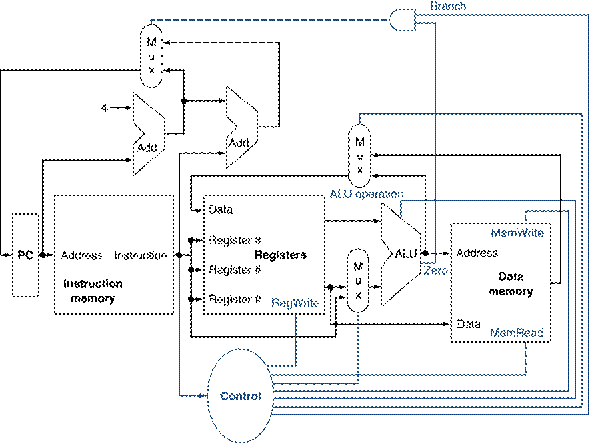Computer Organization and Structure

Homework #3

Due: 2009/12/15

1.      Different instructions utilize different hardware blocks in the basic single-cycle implementation as shown in Figure 1. The next three problems refer to the following instruction:

 Instruction Interpretation a. add Rd, Rs, Rt Reg[Rd]=Reg[Rs]+Reg[Rt] b. lw Rt, Offs(Rs) Reg[Rt]=Mem[Reg[Rs]+Offs]Figure 1: The basic implementation of the MIPS subset, including the necessary multiplexors and control lines.

a.         What are the values of control signals generated by the control in Figure 1 for this instruction?

b.        Which resources (blocks) perform a useful function for this instruction?

c.         Which resources (blocks) produce outputs, but their outputs are not used for this instruction? Which resources produce no outputs for this instruction?

2.      Different execution units and blocks of digital logic have different latencies (time needed to do their work). In Figure 1 there are seven kinds of major blocks. Latencies of blocks along the critical (longest-latency) path for an instruction determine the minimum latency of that instruction. For the following three problems, assume the following resource latencies:

 I-Mem Add Mux ALU Regs D-Mem Control a. 400ps 100ps 30ps 120ps 200ps 350ps 100ps b. 500ps 150ps 100ps 180ps 220ps 1000ps 65ps

a.         What is the critical path for a MIPS AND instruction?

b.        What is the critical path for a MIPS load (LD) instruction?

c.         What is the critical path for a MIPS BEQ instruction?

3.      The basic single-cycle MIPS implementation in Figure 1 can only implement some instructions. New instructions can be added to an existing ISA, but the decision whether or not to do that depends, among other things, on the cost and complexity such an addition introduces into the processor datapath and control. The next three problems refer to this new instruction:

 Instruction Interpretation a. add3 Rd, Rs, Rt,Rx Reg[Rd]=Reg[Rs]+Reg[Rt]+Reg[Rx] b. sll Rt, Rd, Shift Reg[Rd]= Reg[Rt] << Shift (shift left by Shift bits)

a.         Which existing blocks (if any) can be used for this instruction?

b.        Which new functional blocks (if any) do we need for this instruction?

c.         What new signals do we need (if any) from the control unit to support this instruction?

4.      The following problems assume that individual stages of the datapath have the following latencies:

 IF ID EX MEM WB a. 300ps 400ps 350ps 500ps 100ps b. 200ps 150ps 120ps 190ps 140ps

a.         What is the clock cycle time in a pipelined and nonpipelined processor?

b.        What is the total latency of a lw instruction in a pipelined and nonpipelined processor?

c.         If we can split one stage of the pipelined datapath into two new stages, each with half the latency of the original stage, which stage would you split and what is the new clock cycle time of the processor?

5.      The following problems assume that instructions executed by the processor are broken down as follows:

 ALU beq lw sw a. 50% 25% 15% 10% b. 30% 25% 30% 15%

a.         Assuming there are no stalls or hazards, what is the utilization (% of cycles used) of the data memory?

b.        Assuming there are no stalls or hazards, what is the utilization of the write-register port of the “Registers” unit?

c.         Instead of a singl-cycle organization, we can use a multi-cycle organization where each instruction takes multiple cycles but one instruction through stages it actually needs (e.g., ST only takes four cycles because it does not need the WB stage). Compare clock cycle times and execution times with single-cycle, multi-cycle, and pipelined organization.

6.      The following problems refer to the following sequence of instructions:

 Instruction sequence a. lw \$1, 40(\$6) add \$6, \$2, \$2 sw \$6, 50(\$1) b. lw \$5, -16(\$5) sw \$5, -16(\$5) add \$5, \$5, \$5

a.         Indicate dependences and their type.

b.        Assume there is no forwarding in this pipelined processor. Indicate hazards and add nop instructions to eliminate them.

c.         Assume there is full forwarding. Indicate hazards and add nop instructions to eliminate them.

The remaining problems assume the following clock cycle times:

 Without forwarding With full forwarding With ALU-ALU forwarding only a. 300ps 400ps 360ps b. 200ps 250ps 220ps

d.        What is the total execution time of this instruction sequence without forwarding and with full forwarding? What is the speed-up achieved by adding full forwarding to a pipeline that had no forwarding?

e.         Add nop instructions to this code to eliminate hazards if there is ALU-ALU forwarding only (no forwarding from the MEM to the EX stage)?

f.         What is the total execution time of this instruction sequence with only ALU-ALU forwarding? What is the speed-up over a no-forwarding pipeline?